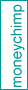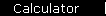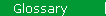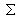### Standard Deviation

A statistical measure of the distance a quantity is likely to lie from its average value. In finance, standard deviation is applied to the annual rate of return of an investment, to measure the investment's volatility, or "risk".

The definition is

StdDev(r) = [1/n *(ri - rave)2]½

where the terms ri are actual values of the investment's annual rate of return, taken over several years, n is the number of values of ri used, and rave is the average value of the ri.

See the article on modern portfolio theory for more details and some examples.

 home  |  glossary  |  calculator  |  about us  |  books